Next: Bidirectional Connections Up: The System Previous: Attention Dynamics

## Recognition Dynamics

Each model cooperates with the image depending on its similarity. The most similar model cooperates most successfully and is the most active one. Hence, the total activity of the model layers indicates which is the correct one. We have derived a winner-take-all mechanism from  evolution equation and applied it to detect the best model and suppress all others. The corresponding equations are (cf. Equations 1 and 7):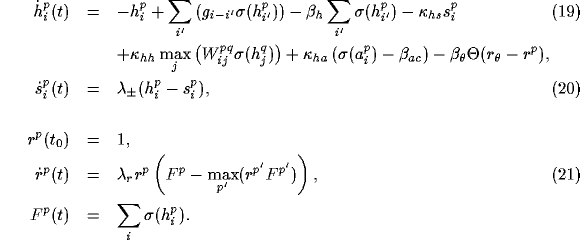The total layer activity is considered as a fitness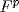, different for each model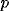. The modified evolution equation can be easily analyzed if theare assumed to be constant in time and the recognition variables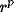are initialized to 1. For the model layer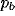with the highest fitness, the equation simplifies to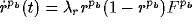with a stable fixed point at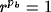. For all other models the equation then simplifies to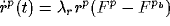, which results in an exponential decay of thefor all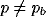. When a recognition variabledrops below the suppression threshold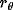, the activity on layeris suppressed by the term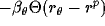. The time scale of the recognition dynamics can be controlled by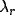.Next: Bidirectional Connections Up: The System Previous: Attention Dynamics